# How to Reverse String using Pointer in C

In my last post I had discussed about how to reverse a string. In this post I am showing another method of reversing a string. I will use a pointer here to reverse the string. In this way I took my input in an character array and then assigned the array into a character type pointer. After that I searched the NULL character using pointer asthmatics. And finally printed the string using pointer in reverse order. Now see the code:

```#include <stdio.h>

int main()
{
char str, *ptr;
int i, len;
printf("Enter a string: ");
gets(str);
ptr = str;
for(i=0;i<1000;i++){
if(*ptr == '\0')  break;
ptr++;
}
len = i;
ptr--;
printf("Reversed String: ");
for(i=len; i>0; i--){
printf("%c",*ptr--);
}
return 0;
}```
Share:

#### 16 comments :

1.2.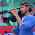Thanks for all programs

3.I Love The site. Please Continue Developeing.

4.This comment has been removed by a blog administrator.

5.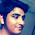6.7.8.Can anyone tell what is wrong with this code "to reverse a string":-

int main()
{
char str[]="abcdefgh";
int i=0;
int n=7;
char temp;
char *ptr;
ptr=str;
while(i<7)
{

temp=ptr[i];
ptr[i]=ptr[n-i];;
ptr[n-i]=temp;

i=i+1;

}
printf("reverse of string is %s\n",str);
}

1.#include

int main()
{
char str[]="abcdefgh";
int i=0;
int n=7;
char temp;
char *ptr;
ptr=str;
while(i<n)
{
temp=ptr[i];
ptr[i]=ptr[n];;
ptr[n]=temp;
i++;
n--;
}
printf("reverse of string is %s\n",ptr);
}

9.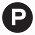;-(
good effort

10.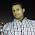#include
int main()
{
char str,str1;
int i,l=0;
printf("Enter any string:");
gets(str);
for(i=0;str[i]!='\0';i++);
for(i--;i>=0;i--)
str1[l++]=str[i];
str1[l]='\0';
printf("reverse of string: %s",str1);
}

11.#include
int main()
{
int i , j, size;
char *str, temp, *ptr;
printf("Enter the size of array:: ");
scanf("%d",&size);
str =(char*)malloc(size* sizeof(char));
printf("Enter the String:: ");
scanf("%s",str);
j = strlen(str) - 1;
for(i = 0;i<=j;i++)
{
temp = str[i];
str[i] = str[j];
str[j] = temp;
j--;
}
printf("\nReverse string is :%s", str);
free(str);
}

This example using runtime memory allocation.

12.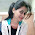13.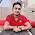C++ Program to Reverse a Strings

Reverse a String means reverse the position of all character of String. You can use swapping concept to reverse any string in c++.

14.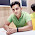Reverse an Array in C++

Nice post thanks for sharing

15.Spam comments will be deleted. :)